DraftKings World Series Odds
+425
4.25 to 1Yankees
16.0% implied probability

+450
4.5 to 1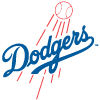Dodgers
15.3% implied probability

+550
5.5 to 1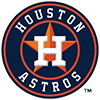Astros
12.9% implied probability

+700
7 to 1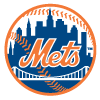Mets
10.5% implied probability

+1100
11 to 1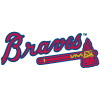Braves
7.0% implied probability

+1200
12 to 1Blue Jays
6.5% implied probability

+1400
14 to 1Padres
5.6% implied probability

+1600
16 to 1Brewers
4.9% implied probability

+3000
30 to 1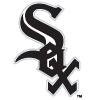White Sox
2.7% implied probability

+3000
30 to 1Giants
2.7% implied probability

+3000
30 to 1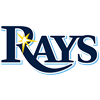Rays
2.7% implied probability

+3000
30 to 1Red Sox
2.7% implied probability

+3500
35 to 1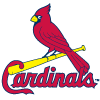Cardinals
2.3% implied probability

+3500
35 to 1Phillies
2.3% implied probability

+4500
45 to 1Twins
1.8% implied probability

+8000
80 to 1Guardians
1.0% implied probability

+9000
90 to 1Angels
0.9% implied probability

+15000
150 to 1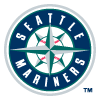Mariners
0.6% implied probability

+35000
350 to 1Marlins
0.2% implied probability

+40000
400 to 1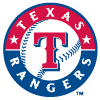Rangers
0.2% implied probability

+100000
1000 to 1Diamondbacks
0.1% implied probability

+100000
1000 to 1Pirates
0.1% implied probability

+100000
1000 to 1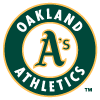Athletics
0.1% implied probability

+100000
1000 to 1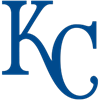Royals
0.1% implied probability

+100000
1000 to 1Tigers
0.1% implied probability

+100000
1000 to 1Rockies
0.1% implied probability

+100000
1000 to 1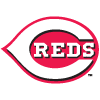Reds
0.1% implied probability

+100000
1000 to 1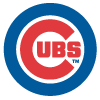Cubs
0.1% implied probability

+100000
1000 to 1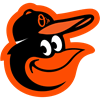Orioles
0.1% implied probability

+100000
1000 to 1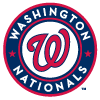Nationals
0.1% implied probability